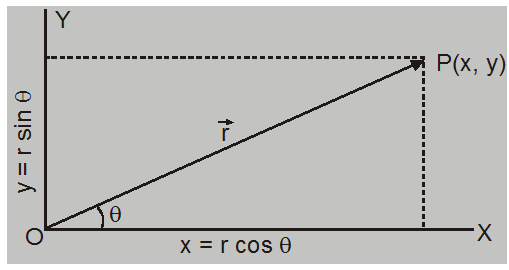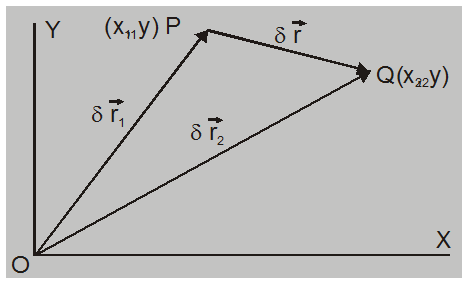Deepak Scored 45->99%ile with Bounce Back Crack Course. You can do it too!

# Motion in two dimensions - Motion in Plane - eSaral

## Motion in Two Dimensions

An object moving in a plane is said to have two-dimensional motion. The two-dimensional motion is equal to the vector sum of two one-dimensional motions along a mutually perpendicular direction.Let the position of point P at a time t be given by position

vector $\overrightarrow{\mathrm{OP}}=\overrightarrow{\mathrm{r}}$

$\vec{r}=\hat{i} r \cos \theta+\hat{j} r \sin \theta$

$=\hat{i} x+\hat{j} y$

## Displacement

Let the position of point P at time $\mathrm{t}_{1}$ be described by position vector $\vec{r}_{1}=x_{1} \hat{i}+y_{1} \hat{j}$ and at time $\mathrm{t}_{2}$

position Q is given by position vector $\vec{r}_{2}=x_{2} \hat{i}+y_{2} \hat{j}$from $\Delta \mathrm{OPQ} \Rightarrow \overrightarrow{\mathrm{OP}}+\overrightarrow{\mathrm{PQ}}=\overrightarrow{\mathrm{OQ}}$

or $\quad \overrightarrow{\mathrm{PQ}}=\overrightarrow{\mathrm{OQ}}-\overrightarrow{\mathrm{OP}}$

Displacement $\overrightarrow{P Q}=\delta \vec{r}=\vec{r}_{2}-\vec{r}_{1}$

in time interval $\delta \mathrm{t}=\left(\mathrm{t}_{2}-\mathrm{t}_{1}\right)$

or

$\vec{\delta}_{r}=\left(x_{2} \hat{i}+y_{2} \hat{j}\right)-\left(x_{1} \hat{i}+y_{1} \hat{j}\right)$

$=\left(x_{2}-x_{1}\right) \hat{i}+\left(y_{2}-y_{1}\right) \hat{j}$

$=\delta \mathrm{x} \hat{\mathrm{i}}+8 \mathrm{y} \hat{\mathrm{j}}$

displacement along X-axis $\delta x=x_{2}-x_{1}$

displacement along Y-axis $\delta y=y_{2}-y_{1}$

Thus, displacement in 2 dimensions is equal to the vector sum of two one dimensional displacements along mutually perpendicular directions.

Let particle move with uniform velocity at $\overrightarrow{\mathrm{V}}$ an angle $\theta$ with X-axis.

Then in component form $\overrightarrow{\mathrm{v}}=\mathrm{v}_{\mathrm{x}} \hat{\mathrm{i}}+\mathrm{v}_{\mathrm{y}} \hat{\mathrm{j}}$

here $v_{x}=v \cos \theta$

and $\quad v_{y}=v \sin \theta$

and $\quad \delta \mathrm{x}=\mathrm{v}_{\mathrm{x}} \delta \mathrm{t}$

$\delta \mathrm{y}=\mathrm{v}_{\mathrm{y}} \delta \mathrm{t}$

or $\delta x=(v \cos \theta) \delta t$

$\delta y=(v \sin \theta) \delta t$

so with $\mathrm{V}_{\mathrm{x}}$ we get displacement along X-axis only and $\mathrm{v}_{\mathrm{y}}$ gives displacement along Y-axis only.

And if particle is moving with uniform acceleration $\overrightarrow{\mathrm{a}}$, then

$\overrightarrow{\mathrm{a}}=\mathrm{a}_{\mathrm{x}} \hat{\mathrm{i}}+\mathrm{a}_{\mathrm{y}} \hat{\mathrm{j}}$

If direction of $\vec{a}$ makes angle $\phi$ with X-axis then $a_{x}=a \cos \phi$ and $\mathrm{a}_{\mathrm{y}}=\mathrm{a} \sin \phi$

are components of $\overrightarrow{\mathrm{a}}$.

Due to $\mathrm{a}_{\mathrm{x}}$, there is a change in the X component of velocity only with no change in Y-component.

Similarly, $\mathrm{a}_{\mathrm{y}}$ will change only the Y component of velocity at time t

So $v_{x}=u_{x}+a_{x} t$

(here $\mathrm{u}_{\mathrm{x}}$ and $\mathrm{u}_{\mathrm{y}}$ are components of initial velocity)

And

$v_{y}=u_{y}+a_{y} t$

Hence

$v_{x} \hat{i}+v_{y} \hat{j}=\left(u_{x}+a_{x} t\right) \hat{i}+\left(u_{y}+a_{y} t\right) \hat{j}$

$=\left(u_{x} \hat{i}+u_{y} \hat{j}\right)+\left(a_{x} \hat{i}+a_{y} \hat{j}\right) t$

Or

$\overrightarrow{\mathrm{v}}=\overrightarrow{\mathrm{u}}+\overrightarrow{\mathrm{a}} \mathrm{t}$

and similarly, component of displacement are

$s_{x}=u_{x} t+\frac{1}{2} a_{x} t^{2}$

And

$s_{y}=u_{y} t+\frac{1}{2} a_{y} t^{2}$

Hence

$s_{x} \hat{i}+s_{y} \hat{j}=\left(u_{x} \hat{i}+u_{y} \hat{j}\right)+\frac{1}{2}\left(a_{x} \hat{i}+a_{y} \hat{j}\right) t^{2}$

Or

$\vec{s}=\vec{u} t+\frac{1}{2} \vec{a} t^{2}$